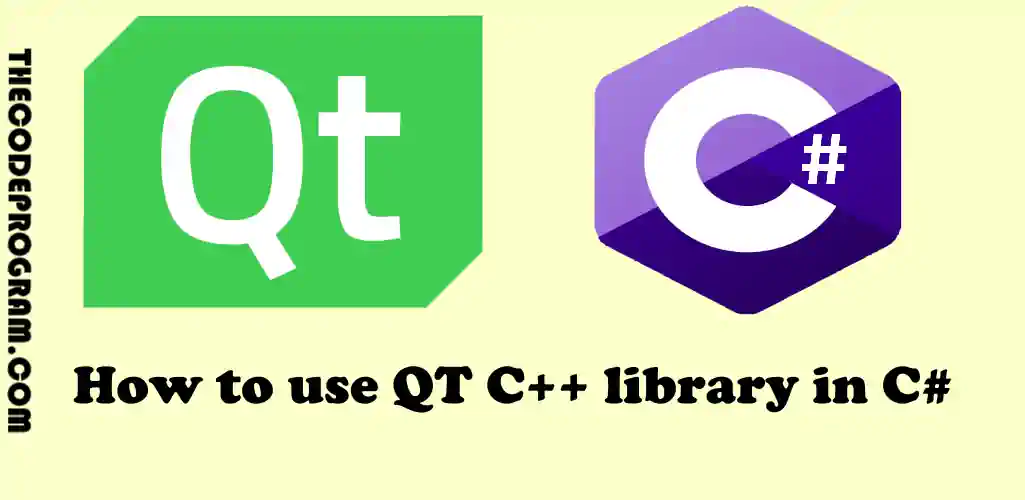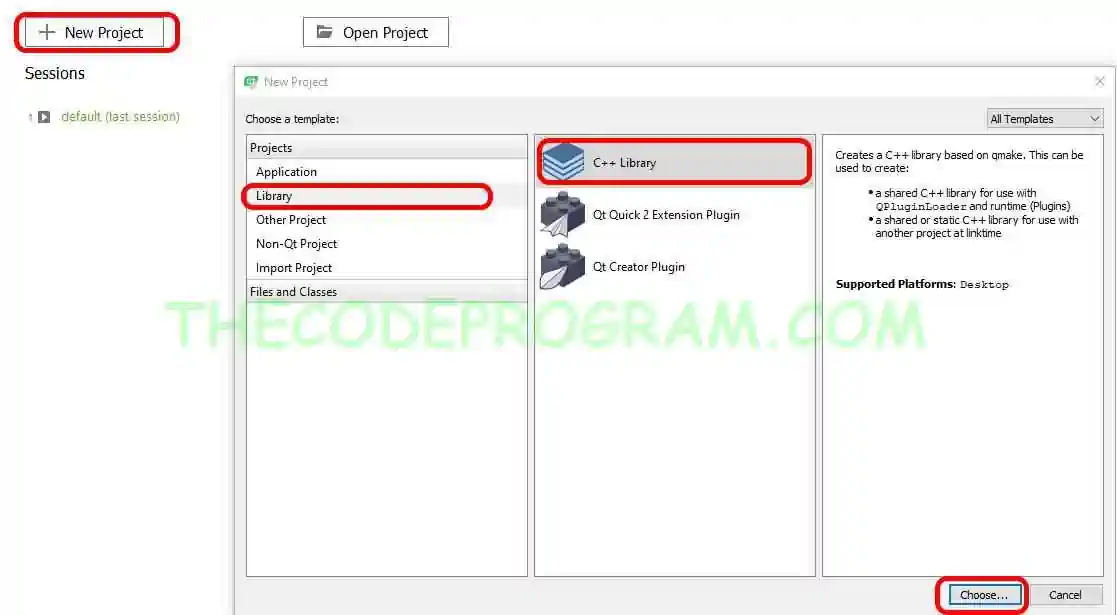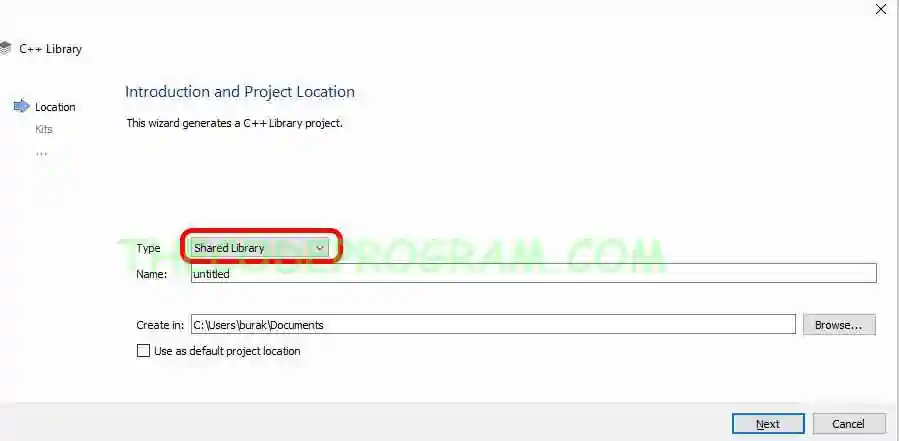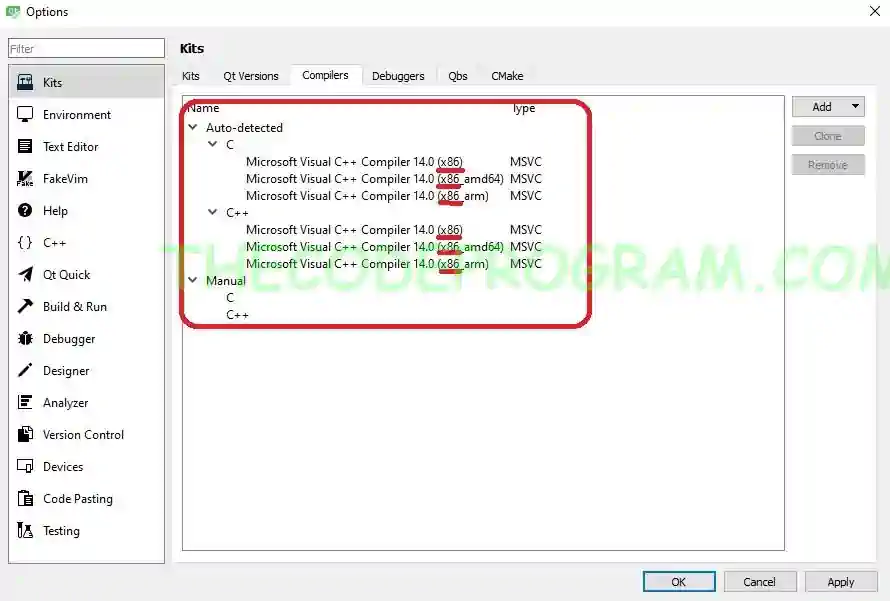## How to use QT C++ library in C#

Hello everyone, in this article we are going to talk about how can we use the libraries which created with QT C++ in our C# applications with Interop Services on an example.

Let's begin.

Firstly we are going to create a library with QT C++. Below image you can see the how to create a library project in QT.And do not forget to select the Shared Library for our library like below image.Now let's write our codes in our library. In here we are going to write some basic mathematical calculations. Below code blocks you will see them.

##### qtlibraryexample.h
``````
#include "qtlibraryexample.h"
#include <stdio.h>

QtLibraryExample::QtLibraryExample()
{
}

extern "C" double add(int a, int b)
{
return a + b;
}

extern "C" double subtrack(int a, int b)
{
return a - b;
}

extern "C" double multiply(int a, int b)
{
return a * b;
}

extern "C" QTLIBRARYEXAMPLESHARED_EXPORT double divide(int a, int b)
{
return a / b;
}
``````
##### qtlibraryexample_global.h
``````
#ifndef QTLIBRARYEXAMPLE_GLOBAL_H
#define QTLIBRARYEXAMPLE_GLOBAL_H

#include <QtCore/qglobal.h>

#if defined(QTLIBRARYEXAMPLE_LIBRARY)
#  define QTLIBRARYEXAMPLESHARED_EXPORT Q_DECL_EXPORT
#else
#  define QTLIBRARYEXAMPLESHARED_EXPORT Q_DECL_IMPORT
#endif

extern "C" __declspec(dllexport) double add(int a, int b);
extern "C" __declspec(dllexport) double subtrack(int a, int b);
extern "C" __declspec(dllexport) double multiply(int a, int b);
extern "C" __declspec(dllexport) double divide(int a, int b);

#endif // QTLIBRARYEXAMPLE_GLOBAL_H
``````
And lastly Build the project. Do not try to run this project just Build it to create dll files.
Also do not forget our library is 32bit. We are going to need this information in our C# program. Below image you can see the QT library compiler is compiling 32 bit.We will activate the 32bit api usage in Visual Studio project properties.
Now we creat a C# application in MS Visual Studio.
First we are going to include Interop Services in our C# project.
``````
using System.Runtime.InteropServices;
``````
And then we are going to define the prototype methods which we defined in our C++ library. These methods must be same as the defined in C++ library.
And then we are going to create the function which will call these methods.
``````
[DllImport("QtLibraryExample.dll")]
static extern double add(int a, int b);

[DllImport("QtLibraryExample.dll")]
static extern double subtrack(int a, int b);

[DllImport("QtLibraryExample.dll")]
static extern double multiply(int a, int b);

[DllImport("QtLibraryExample.dll")]
static extern  double divide(int a, int b);

static void callQtFunctions()
{
Console.WriteLine("Result of 5 + 7 is " + add(5, 7).ToString());
Console.WriteLine("Result of 21 - 10 is " + subtrack(21, 10).ToString());
Console.WriteLine("Result of 9 x 3 is " + multiply(9, 3).ToString());
Console.WriteLine("Result of 70 / 5 is " + divide(70, 5).ToString());
}
``````
All we have to do call this method in main function.
``````
static void Main(string[] args)
{
Console.Title = "Using QT Libraries in C# Aplication Example - TheCodeProgram";
callQtFunctions();
}
``````
Now I copied all of QT library files into visual studio executable file folder. This is important.
Also set the project as Release mode and 32-bit. Because our library has been built as 32bit as showed above. Below image you can see the 32bit building of Visual Studio project property.

You can reach the example application on Github via : https://github.com/thecodeprogram/QtLibraryForCSharpApplication

Have a good managing unmanaged codes.

Burak Hamdi TUFAN.

### FROM : General Programming

17/10/2020

29/10/2020

04/09/2023

#### How to use Vector in Java

• Soleimani

Hi. How can show a QDialog from .dll in c#?

2021/05/31 18:54:55
• Burak Hamdi TUFAN

Hello, If I am not wrong, you only use data types which compatible in both structure. For Example in this example we used double type. Double type is primitive and compatible in both languages.
I believe you can not show a QDialog directly in C# program because of this problem. But if you are in need to show and it is essential you can create a simple QWidget application which dedicated to show a QDialog, you can start it from your C# program. Also you can send parameter at starting and you can perform specified operations.

2021/06/16 21:58:52
• Soleimani

Thank you very much.

2021/06/17 09:21:58